# Breadth-first search traversal in Javascript

BFS visits the neighbor vertices before visiting the child vertices, and a queue is used in the search process. Following is how a BFS works −

• Visit the adjacent unvisited vertex. Mark it as visited. Display it. Insert it in a queue.
• If no adjacent vertex is found, remove the first vertex from the queue.
• Repeat Rule 1 and Rule 2 until the queue is empty.

Let us look at an illustration of how BFS Traversal works:

Step
Traversal
Description
1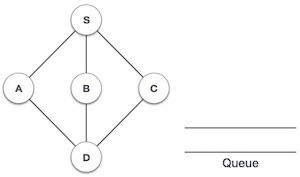Initialize the queue.
2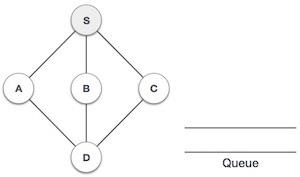We start by visiting S (starting node) and mark it as visited.
3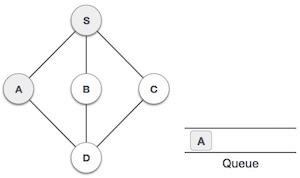We then see an unvisited adjacent node from S. In this example, we have three nodes but alphabetically we choose A, mark it as visited and enqueue it.
4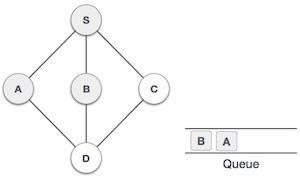Next, the unvisited adjacent node from S is B. We mark it as visited and enqueue it.
5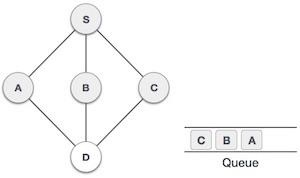Next, the unvisited adjacent node from S is C. We mark it as visited and enqueue it.
6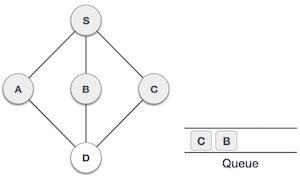Now, S is left with no unvisited adjacent nodes. So, we dequeue and find A.
7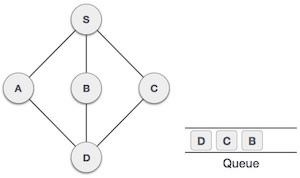From  A we have as an unvisited adjacent node. We mark it as visited and enqueue it.

At this stage, we are left with no unmarked (unvisited) nodes. But as per the algorithm we keep on dequeuing in order to get all unvisited nodes. When the queue gets emptied, the program is over.

Let's look at how we can implement this in JavaScript.

## Example

BFS(node) {
// Create a Queue and add our initial node in it
let q = new Queue(this.nodes.length);
let explored = new Set();
q.enqueue(node);

// Mark the first node as explored explored.

// We'll continue till our queue gets empty
while (!q.isEmpty()) {
let t = q.dequeue();

// Log every element that comes out of the Queue
console.log(t);

// 1. In the edges object, we search for nodes this node is directly connected to.
// 2. We filter out the nodes that have already been explored.
// 3. Then we mark each unexplored node as explored and add it to the queue.
this.edges[t]
.filter(n => !explored.has(n))
.forEach(n => {
q.enqueue(n);
});
}
}

You can test this function using −

## Example

let g = new Graph();

g.BFS("A");

## Output

This will give the output −

A
C
B
D
G
E
F